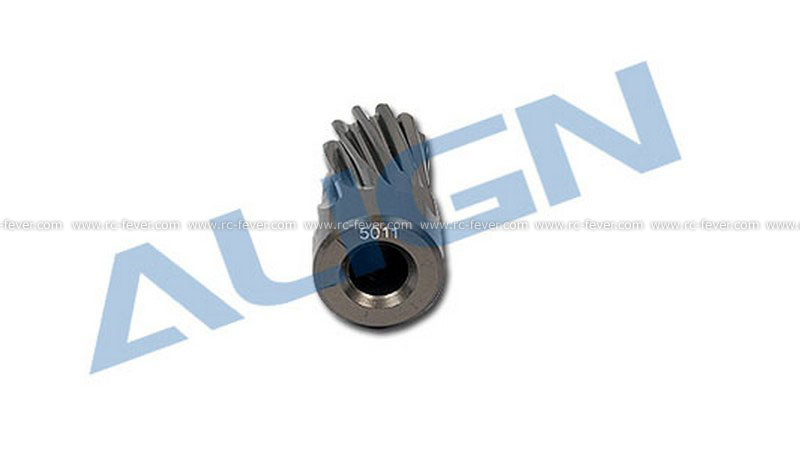# What is the Gear Ratio and How it Affect your Helicopter?

Gear is very useful in the daily life, for example the car engine use the gear to allow high torque at the beginning and high speed when driving.Gear can change the rotating speed, adding the gear structure in the RC model is a common practice. Take RC helicopter as an example, due to the ratio different of gears, it allow use to use the small motor to move a big blade. Fine tuning the gear ratio can change the torque and rotating speed, allow the motor rotating in the high efficient speed and push the blade to rotate in the optimums speed.

Gear ratio: How to calculate the gear ratio? It is very easy. Using the number of tooth of the bigger gear, divide the number of teeth of the smaller gear. For example the bigger gear is 150T, smaller gear is 13T. The gear ratio is 150 divided by 13, about 11.53. It means when the small gear rotate 11.53 times, the bigger gear rotate for one circle. Therefore the higher gear ratio, the more torque and the lower rotating speed it produce.How to set the optimums gear ratio? Take a 450 class helicopter with the 3500kv motor as an example. Use the 11.1V Li-Po battery. 3500 multiple 11.1 is 39960rpm, with the gear ratio 11.53. The blade rotating speed is 39960 divide 11.53 equal to 3466. 3466 is already okay for beginner, but if you want a higher rotating speed of blade, you can change the higher number of tooth of gear let say 14T. But you need to take good care on this ratio, if you want more rotating speed, the torque will become lower and if the motor have not enough torque it will harm the motor.

Helicopter gear ratio expression: Take a 450 class helicopter as an example, the rotating parts are motor, main blade and tail blade, the ratio expression just like this: 11.53:1:4. 150/13=11.53, 1:4 is the ratio between main blade and tail blade, when the main blade rotate for one time, the tail blade will rotate for 4 times. This ratio can be calculated by the number of tooth of tail gear group.